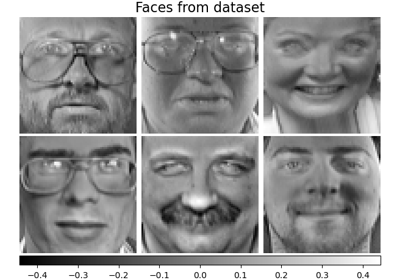# sklearn.decomposition.MiniBatchSparsePCA¶

class sklearn.decomposition.MiniBatchSparsePCA(n_components=None, *, alpha=1, ridge_alpha=0.01, n_iter=100, callback=None, batch_size=3, verbose=False, shuffle=True, n_jobs=None, method='lars', random_state=None)[source]

Mini-batch Sparse Principal Components Analysis

Finds the set of sparse components that can optimally reconstruct the data. The amount of sparseness is controllable by the coefficient of the L1 penalty, given by the parameter alpha.

Read more in the User Guide.

Parameters
n_componentsint, default=None

number of sparse atoms to extract

alphaint, default=1

Sparsity controlling parameter. Higher values lead to sparser components.

ridge_alphafloat, default=0.01

Amount of ridge shrinkage to apply in order to improve conditioning when calling the transform method.

n_iterint, default=100

number of iterations to perform for each mini batch

callbackcallable, default=None

callable that gets invoked every five iterations

batch_sizeint, default=3

the number of features to take in each mini batch

verboseint or bool, default=False

Controls the verbosity; the higher, the more messages. Defaults to 0.

shufflebool, default=True

whether to shuffle the data before splitting it in batches

n_jobsint, default=None

Number of parallel jobs to run. None means 1 unless in a joblib.parallel_backend context. -1 means using all processors. See Glossary for more details.

method{‘lars’, ‘cd’}, default=’lars’

lars: uses the least angle regression method to solve the lasso problem (linear_model.lars_path) cd: uses the coordinate descent method to compute the Lasso solution (linear_model.Lasso). Lars will be faster if the estimated components are sparse.

random_stateint, RandomState instance or None, default=None

Used for random shuffling when shuffle is set to True, during online dictionary learning. Pass an int for reproducible results across multiple function calls. See Glossary.

Attributes
components_ndarray of shape (n_components, n_features)

Sparse components extracted from the data.

n_components_int

Estimated number of components.

New in version 0.23.

n_iter_int

Number of iterations run.

mean_ndarray of shape (n_features,)

Per-feature empirical mean, estimated from the training set. Equal to X.mean(axis=0).

Examples

>>> import numpy as np
>>> from sklearn.datasets import make_friedman1
>>> from sklearn.decomposition import MiniBatchSparsePCA
>>> X, _ = make_friedman1(n_samples=200, n_features=30, random_state=0)
>>> transformer = MiniBatchSparsePCA(n_components=5, batch_size=50,
...                                  random_state=0)
>>> transformer.fit(X)
MiniBatchSparsePCA(...)
>>> X_transformed = transformer.transform(X)
>>> X_transformed.shape
(200, 5)
>>> # most values in the components_ are zero (sparsity)
>>> np.mean(transformer.components_ == 0)
0.94


Methods

 fit(X[, y]) Fit the model from data in X. fit_transform(X[, y]) Fit to data, then transform it. get_params([deep]) Get parameters for this estimator. set_params(**params) Set the parameters of this estimator. Least Squares projection of the data onto the sparse components.
fit(X, y=None)[source]

Fit the model from data in X.

Parameters
Xarray-like of shape (n_samples, n_features)

Training vector, where n_samples in the number of samples and n_features is the number of features.

yIgnored
Returns
selfobject

Returns the instance itself.

fit_transform(X, y=None, **fit_params)[source]

Fit to data, then transform it.

Fits transformer to X and y with optional parameters fit_params and returns a transformed version of X.

Parameters
Xarray-like of shape (n_samples, n_features)

Input samples.

yarray-like of shape (n_samples,) or (n_samples, n_outputs), default=None

Target values (None for unsupervised transformations).

**fit_paramsdict

Additional fit parameters.

Returns
X_newndarray array of shape (n_samples, n_features_new)

Transformed array.

get_params(deep=True)[source]

Get parameters for this estimator.

Parameters
deepbool, default=True

If True, will return the parameters for this estimator and contained subobjects that are estimators.

Returns
paramsdict

Parameter names mapped to their values.

set_params(**params)[source]

Set the parameters of this estimator.

The method works on simple estimators as well as on nested objects (such as Pipeline). The latter have parameters of the form <component>__<parameter> so that it’s possible to update each component of a nested object.

Parameters
**paramsdict

Estimator parameters.

Returns
selfestimator instance

Estimator instance.

transform(X)[source]

Least Squares projection of the data onto the sparse components.

To avoid instability issues in case the system is under-determined, regularization can be applied (Ridge regression) via the ridge_alpha parameter.

Note that Sparse PCA components orthogonality is not enforced as in PCA hence one cannot use a simple linear projection.

Parameters
Xndarray of shape (n_samples, n_features)

Test data to be transformed, must have the same number of features as the data used to train the model.

Returns
X_newndarray of shape (n_samples, n_components)

Transformed data.

## Examples using sklearn.decomposition.MiniBatchSparsePCA¶